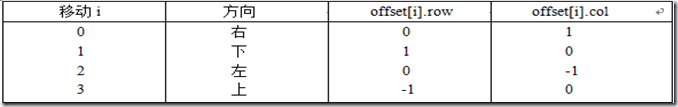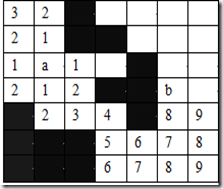基础算法系列总结：分支限界算法

（1） 定义一个表示电路板上方格位置的类Position。（2） 用二维数组grid表示所给的方格阵列。View Code
#include <stdio.h>
typedef
struct {

int row;

int col;
}Position;
int FindPath (Position start, Position finish, int &PathLen, Position *&path)
//计算从起始位置start到目标位置finish的最短布线路径，找到返回1，否则，返回0
int  i;

if ((start.row = = finish.row) && (start.col = = finish.col)) {
PathLen
= 0;   return 0; } //start = finish

//设置方格阵列”围墙”
for (i = 0; i <= m+1; i++)
grid[
0][i] = grid[n+1][i] = 1//顶部和底部
for (i = 0; i <= n+1; i++)
grid[i][
0= grid[i][m+1= 1//左翼和右翼

//初始化相对位移
int  NumOfNbrs = 4//相邻方格数
Position offset, here, nbr;
offset[
0].row = 0;   offset.col = 1;   //
offset.row = 1;   offset.col = 0;   //
offset.row = 0;   offset.col = -1;  //
offset.row = -1;  offset.row = 0;  //
here.row = start.row;
here.col
= start.col;
<Position> Q; //标记可达方格位置
do {
for (i = 0; i< NumOfNbrs; i++) { //标记可达相邻方格
nbr.row = here.row + offset[i].row ;
nbr.col
= here.col + offset[i].col;
if (grid[nbr.row][nbr.col] = = 0) { //该方格未标记
grid[nbr.row][nbr.col] = grid[here.row][here.col] + 1;
if ((nbr.row = = finish.row) && (nbr.col = = finish.col))  break;//完成布线
}
}
if ((nbr.row = = finishi.row) && (nbr.col = = finish.col))  break;//完成布线
if (Q.IsEmpty()) //活队列是否为空
return 0//无解
Q.delete(here); //取下一个扩展结点
}while (1);
//构造最短布线路径
PathLen = grid[finish.row][finish.col] － 2;
path
= new Position[PathLen];
here
= finish;
for (int j = PathLen – 1; j >= 0; j--) { //找前驱位置
path[j] = here;

for (i = 0; i< NumOfNbrs; i++) {
nbr.row
= here.row + offset[i].row ;
nbr.col
= here.col + offset[i].col;
if (grid[nbr.row][nbr.col] = = j+2)  break;
}
here
= nbr; //向前移动
}
return 1;
}
void main ()
{

int grid;

int PathLen, *path;
Position start, finish;
start.row
= 3;  start.col = 2;
finish.row
= 4; finish.col = 6;

FindPath (start, finish, PathLen, path);
}

• 分支限界法常以广度优先或以最小耗费（最大效益）优先的方式搜索问题的解空间树。

• 在分支限界法中，每一个活结点只有一次机会成为扩展结点。活结点一旦成为扩展结点，就一次性产生其所有儿子结点。

• 在这些儿子结点中，导致不可行解或导致非最优解的儿子结点被舍弃，其余儿子结点被加入活结点表中。

• 此后，从活结点表中取下一结点成为当前扩展结点，并重复上述结点扩展过程。这个过程一直持续到找到所需的解或活结点表为空时为止。

（1）求解目标不同：回溯法的求解目标是找出解空间树中满足约束条件的所有解，而分支限界法的求解目标则是找出满足约束条件的一个解，或是在满足约束条件的解中找出在某种意义下的最优解。

（2）搜索方式的不同：回溯法以深度优先的方式搜索解空间树，而分支限界法则以广度优先或以最小耗费优先的方式搜索解空间树。

算法系列目录：

posted @ 2011-05-21 19:46  大熊先生|互联网后端技术  阅读(4208)  评论(1编辑  收藏  举报